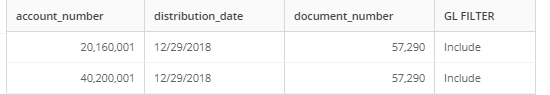# Case Statement with AND function in SQL

I am using below query to mark the two fileds as 'Exclude'

SELECT *, CASE WHEN `account_number` = 20160001 and `document_number` = 57290 and `distribution_date` = '12/29/2018' then 'exclude'
WHEN `account_number` = 40200001 and `document_number` = 57290 and `distribution_date` = '12/29/2018' then 'exclude'
else 'Include' end as `GL FILTER` from `table`

However, I get the following result: the GL FIlter is 'Include' I want it to be 'Exclude'Thanks,

• I'm going to guess the issue has to do with your date column. If distribution date is seen as a date field and not text, you need to update your conditions to look like this:

`SELECT *, CASE WHEN `account_number` = 20160001 and `document_number` = 57290 and `distribution_date` = '2018-12-29' then 'exclude'WHEN `account_number` = 40200001 and `document_number` = 57290 and `distribution_date` = '2018-12-29' then 'exclude'else 'Include' end as `GL FILTER` from `table``

If it's a datetime, then you'll need to format for that instead.

Hopefully that gets you what you're after.

Best of luck,

Valiant